# Excel Array Formulas

These are formulas designed to apply to single or multiple items at a single course of actionReviewed by

Rohan Arora

Expertise: Investment Banking | Private Equity

Updated:

August 11, 2023

Excel has various tools and features, making it one of the most used and required tools for corporations.

Of the available features, the popular ones are the functions and formulas. These features allow the user to solve various problems with a single code or syntax, avoid complex issues or errors and design the worksheets orderly.

However, one of the salient features of formulas is customization, i.e., setting up your criteria and conditions and arriving at the outcomes desired. Isn't that what recipes are for?

We will input some logic in the syntax in a way that makes the software easy to interpret and provides us with the results.

The features, tools, and shortcut keys are built on formulas, which are logical algorithms. So, the most commonly used procedures like sum, count, and average are implemented with just a click; for other formulas, the user needs to follow the process and shortcuts to execute their criteria.

You might have heard about financial, logical, textual, date and time, lookup and reference, math & trigonometric and statistical, and many formulas while looking at the excel interface.

And you are probably wondering where these formulas were present. Don't worry; we will look at these formulas in detail, along with examples, to better understand.

## Types of Array Formulas

An array is a collection of numerous items of the same data type. Since an array deals with similar things (data), array formulas are formulas designed to apply to single or multiple items at a single course of action.

It is challenging to perform any logical or mathematical operation on multiple items. But, these formulas are primarily known for performing complicated calculations on single or multiple elements.

That being said, an array formula is different from regular formulas in excel, as it focuses on all the values of the selected range and performs numerous complicated calculations by the conditions set.

The data type of items can be text or numbers in rows or columns and multiple rows and columns. So, your data is scattered across the sheet, and you are organizing it with the array formula's help.

A single array formula results in a single output within one cell while performing multiple calculations. We also have a numerous array algorithm that intakes multiple values simultaneously and results in an array. Further, we will look at examples to have more understanding.

We knew that a regular formula does not apply to multiple items and is incompetent in performing complex calculations. To address this loophole, these formulas were designed.

1. Regular Formula

Problem Statement: To find the maximum of marks obtained by a student.

We are provided with the marks for two subjects. First, we find the total defects by using the sum formula.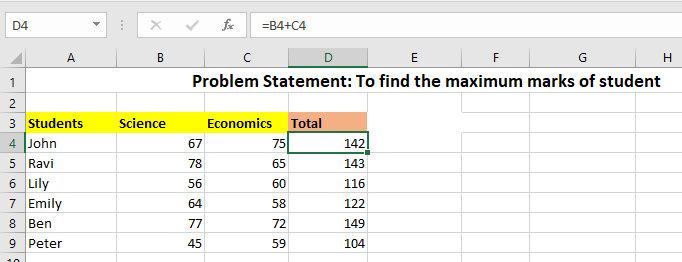We will use the MAX function to find the maximum or the highest value from the total calculated.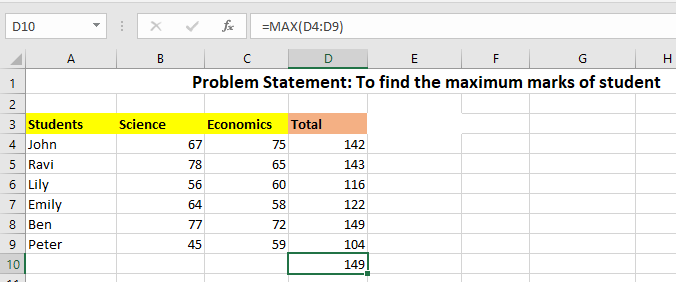2. Array Formula

Using an array algorithm, we can apply the two steps in a single formula.

1. Find the maximum of the two subjects using the MAX function.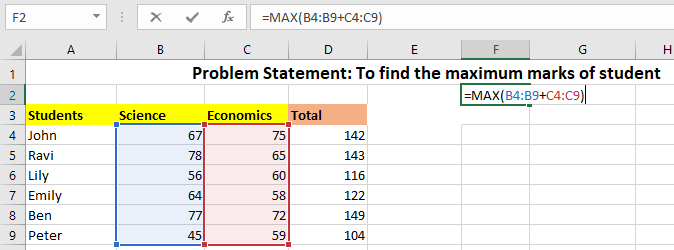2. Press Ctrl+ Shift+ Enter. This is a shortcut key to execute the array algorithm. We can also observe the changes in the formula tab; a square bracket is seen, which means excel recognized the array, which prompted us with the changes.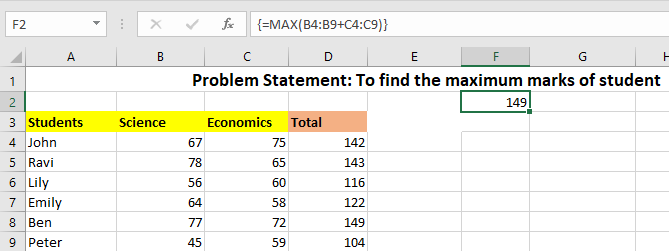## Understanding Array Formulas in Microsoft Excel

Excel does have a special syntax, which helps the software and users recognize the array algorithm.

Don't be frightened; this is simple. For all the versions of Microsoft, such8 as Office 2010, Office2013, Office 2016, Office 2019, and earlier, we have a shortcut key to indicate an array.

That shortcut key is ctrl+ shift+ enter (CSE). This shortcut is also called as CSE formula. Instead of pressing the "Enter" button, we need to press this shortcut key in all the versions of Office except Excel 365 to apply the formula correctly.

Curly brackets will appear in the formula bar as soon as you enter the shortcut key, indicating it is an array formula.

So, in a sense, the syntax would be in the format of {formula}.

## Note

Do not enter the curly braces manually. These are prompted automatically as soon as excel recognizes the algorithm.

However, there are some exceptions also. For example, a few formulas don't need the shortcut key for application and can be entered natively, i.e., by pressing the ENTER button. One such example is the case of the SUMPRODUCT function, which can be entered natively.

Let's take a look at the steps to apply the formula:

We will look at the steps to be followed to complete the formulation of an array. The steps are explained only to provide feasibility to the reader. Go through the steps thoroughly to have more context.

1. Application of formula

Excel has many procedures, and these formulas are applied according to the users' requirements. So, these formulas input various recipes, which are feasible for users to arrive at the task faster.
Some popular formulas inputted are lookups, if conditions, sumif, sum, max, min, and so on.

2. After inputting the formula, press ctrl+shift+enter. You have completed the necessary action. The curly brackets can be seen in the formula bar, which implies the formula is an array formula.

## Note

• If you enter the curly brackets by typing, the array formula will not be recognized by excel.
• The curly brackets will disappear whenever you edit the formula, and you must press the shortcut key again to input the procedure as an array one.
• An array formula is standard unless and until the shortcut key is injected.

## Single Cell Array and Multiple Cell Array

These formulas are the most popular way of incorporating multiple criteria. However, they also deal with a single bar. Let us understand the single and various criteria with examples.

1. Single Cell Array or Single Criteria

Whether the array is a single-cell array or multiple cells, one will be dependent on the result. If the influence is spread in a single cell, it is a single-cell array.

Case: To find the maximum marks obtained.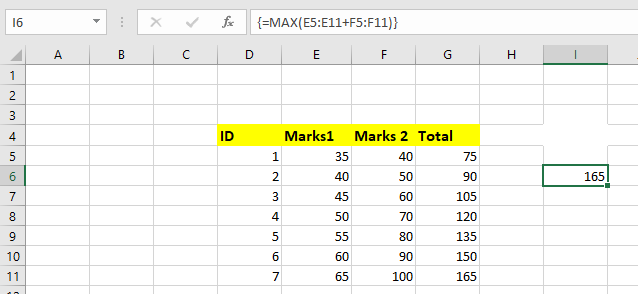To find the maximum marks, we use the entire function. The result is restricted to a single cell and can also be seen in the cell above. Look at the multiple-cell array to get more context.

2. Multiple Cell Array

An array is called a multiple-cell array because the results are not restricted to a single cell and can accurately display the results in multiple cells. These formulas are often considered multiple cells because we will get results simultaneously instead of a single output.

So, the tool has become more popular as it saves time and ensures efficiency.

For example, if you want to find an employee's name, department, and designation at a time, the picture of multiple arrays comes in. Refer to the first example in the examples of array formulas. We also have Dynamic array formulas, which are an extension of the normal ones.

## Excel Array Formulas Examples

Let us understand these formulas in detail using various functions.

Example #1: Array Formula in Excel using VLOOKUP

Case: When you want to apply the lookup function to multiple cells.

Indeed, this combination is a game changer when identifying the values from a large data set.

Let us follow the procedure to execute the VLOOKUP function.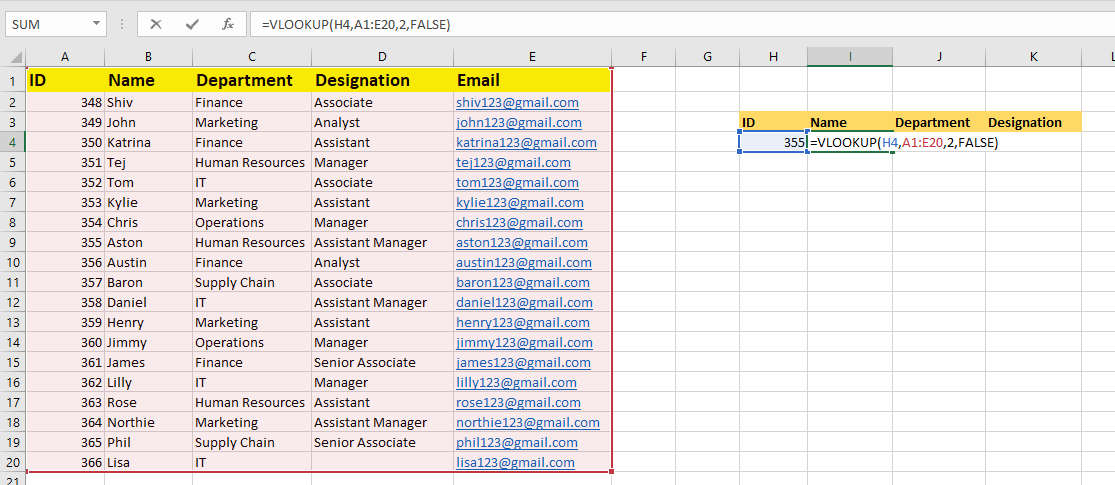If you want to find the lookup data of Name, Department, and Designation at a time, the array and lookup function comes into the process. So let's see how we can do it.

Step 1: Since we want to determine the values of name, department, and designation, select the cells at a time.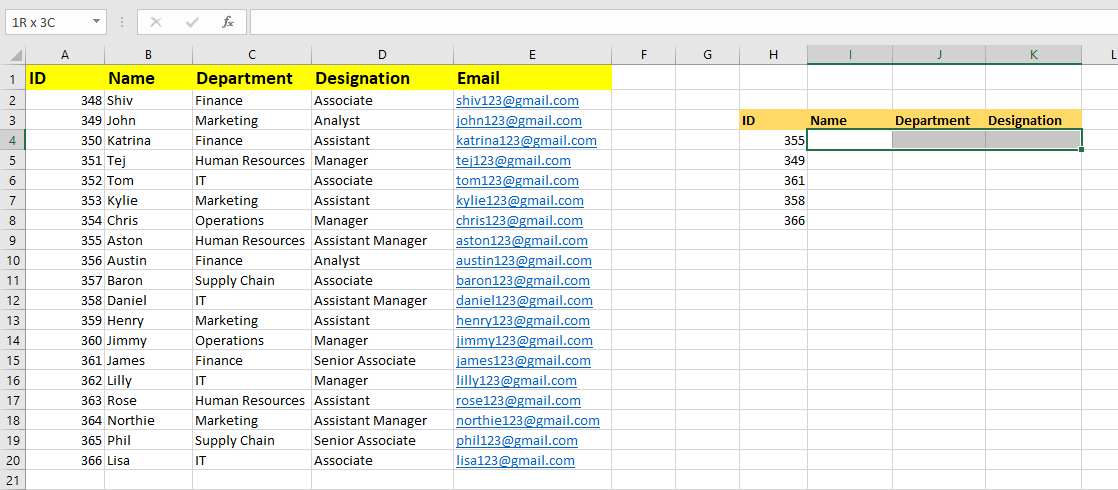Step 2: Now, start entering the lookup formula. Carefully follow the syntax. In this case,

lookup_value= 355, table_array= select the table range, col_index_num= {2,3,4} since we want to get data into three columns and [range_lookup]= false. We are done with the lookup formula.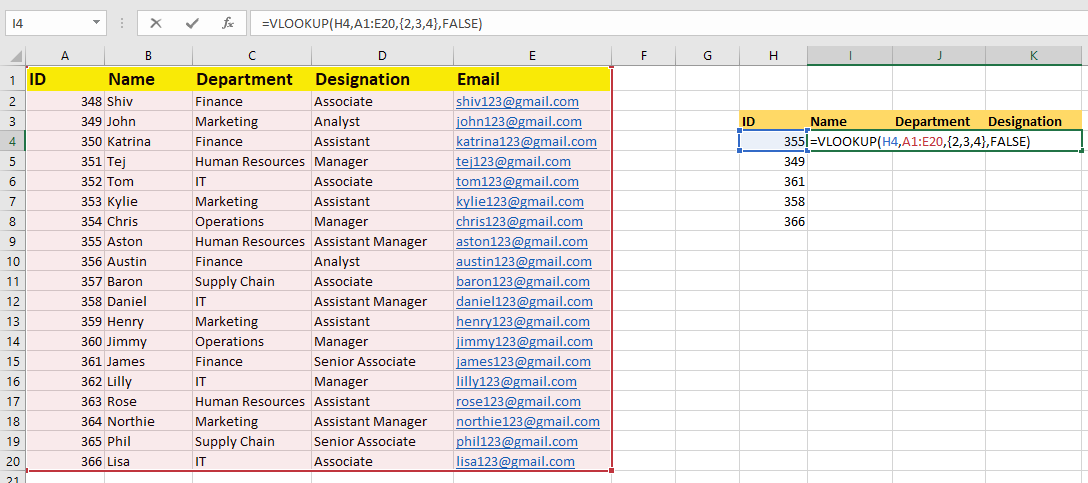Step 3: This is the most crucial step, where we will press ctrl+shift+enter instead of just entering to convert the lookup formula into an array formula.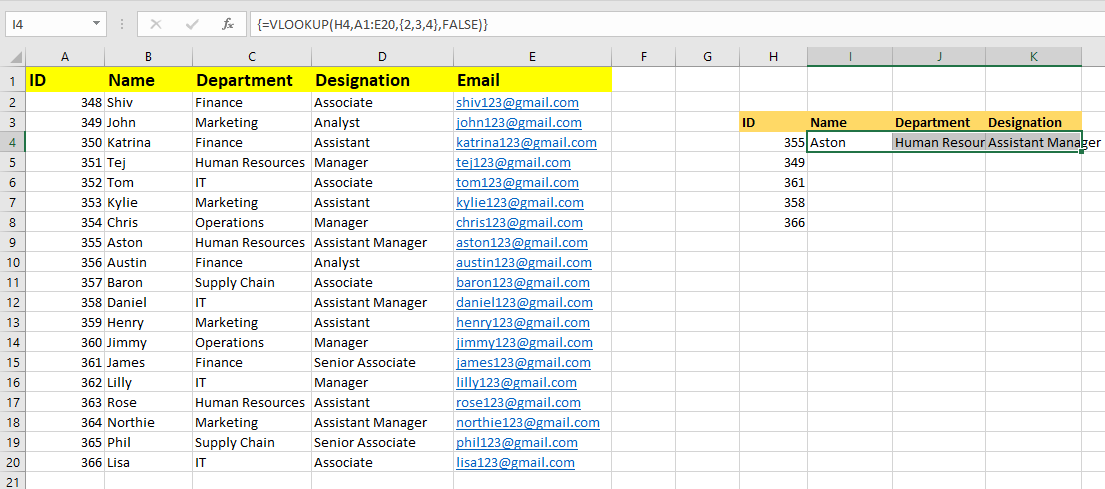That's it; we can see in the formula bar that the appearance of curly brackets converts the lookup formula.Example #2: Array Formula using IF function

Case: Find the maximum quantity of Red Shirts Sold. The source data is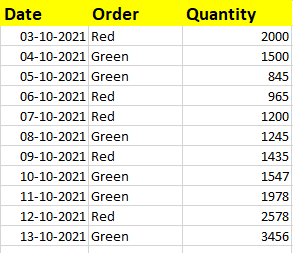Step 1: Application of MAX function. Ultimately, we want the maximum quantity of red shirts.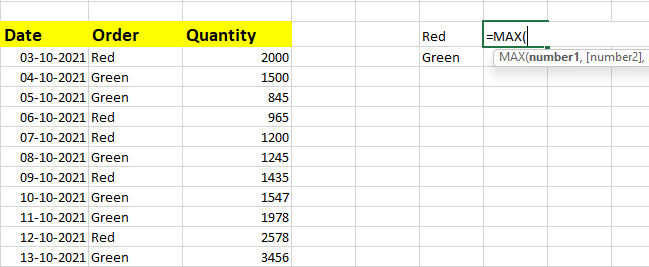Step 2: Now, we use the IF function to precisely get the highest quantity of Red Shirts.

The syntax goes logical test = select the cell last, i.e., Red and red= select the range of order column.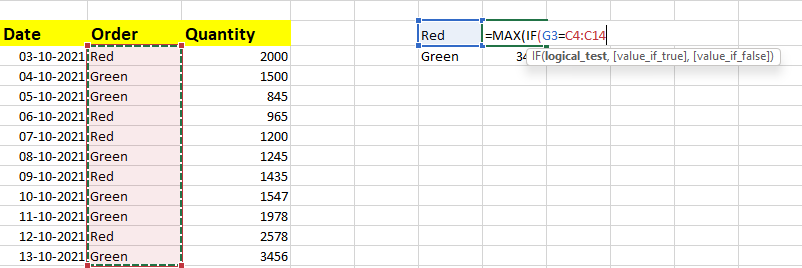Step 3: The next step in the syntax is value_if_true= select the quantity range. Since we want the highest quantity of red ones, ignore value_if_false or give any number because we are not concerned with it.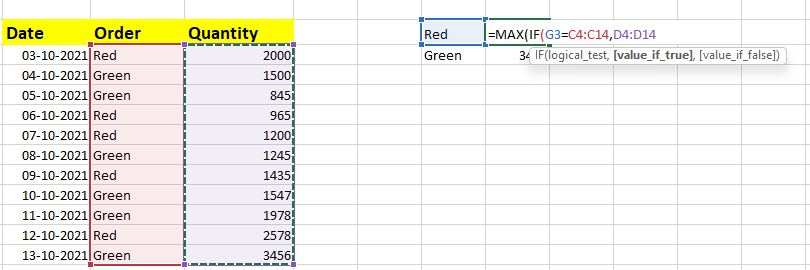Step 4: Close the brackets. Press ctrl+shift+enter.## Importance of Array Formulas

We have seen how array formulas work along with the examples. Let us also examine the importance of arrays and why these array formulas are popular.

1. Saves Time

Since arrays deal with applying formulas on multiple items, we can save time. The amount of time spent using the array formula is the same as a normal function or procedure.

However, the array formula can incorporate multiple criteria, which is not the case with recipes.

2. Reduces complexity

Entering a formula for every criterion is very tedious when dealing with spreadsheets. However, we can select single or multiple criteria at once using the procedures of the array.

We will reduce the complexity by selecting multiple criteria at once and attain the required and accurate results. Formulas are created to reduce complexity, but these array formulations make things easier.

Excel, the powerful tool itself, was designed to reduce complexity by solving problems at a faster time. These multiple-cell criteria features will unravel the complexities at a quicker pace.

3. Ensures Flexibility

Since the usual formula and array go hand in hand, it becomes easy for the user to understand and implement them. And also, with just a shortcut key, i.e., CSE (Ctrl+Shift+Enter), we will be converting the average formula into an array one; isn't it flexible?

4. Accuracy

Accuracy is questionable at times when dealing with large amounts of data. However, thanks to the array of formulas, we can look at the procedure entered with multiple criteria to ensure that the data is accurate and reliable.

5. Error Handling

Though this tool is not directly related to preventing errors, it deals with the non-occurrence of mistakes. In addition, since we deal with multiple criteria in a single formula, it helps reduce errors.

## Key Takeaways

• A regular formula will not execute multiple results simultaneously, so these array formulas are designed and implemented to solve the problem.
• It is elementary for Excel to input and recognizes these formulas; It also helps users to realize that the usual procedure is converted into one formula by a mere curly bracket.
• We can operate on a single cell or multiple cells according to our requirements.
• We have also seen various examples of how the tool is flexible to collaborate with other Excel functions, reducing complexity.
• The CSE shortcut key is not applicable in the case of Excel 365 and the Excel 2021 version. But just pressing the Enter key would finish the task.
• You will finish your work with a single click (CSE), making the next task easy.

### Excel Array Formulas FAQs